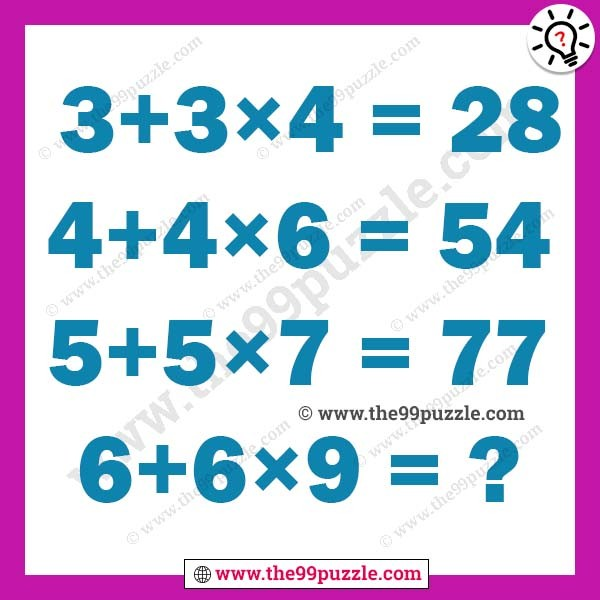# Maths logic problem for adults – Puzz121

In these types of maths logic problem for adults and kids. Here is the Brain Teaser which will test your math skills as well as logical reasoning power. In this math puzzles, some logical equations are given. Can you find the logic in these equations? Let us see how much time you will take to solve this maths logic problem for adults equation?

3+3×4=28

4+4×6=54

5+5×7=77

6+6×9=?###### Explanation:

3+3×4=(3×4)+(3×4)+4=28

4+4×6=(4×6)+(4×6)+6=54

5+5×7=(5×7)+(5×7)+7=77

6+6×9=(6×9)+(6×9)+9=117